Ex 5.3

Chapter 5 Class 10 Arithmetic Progressions
Serial order wise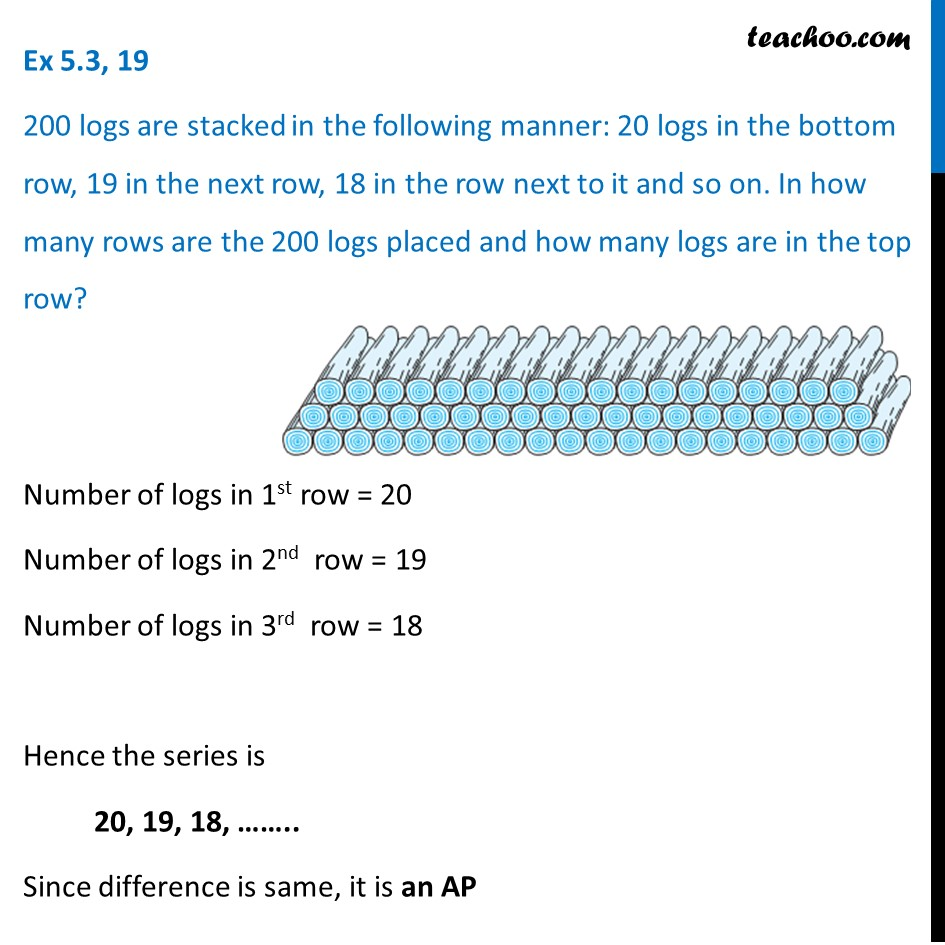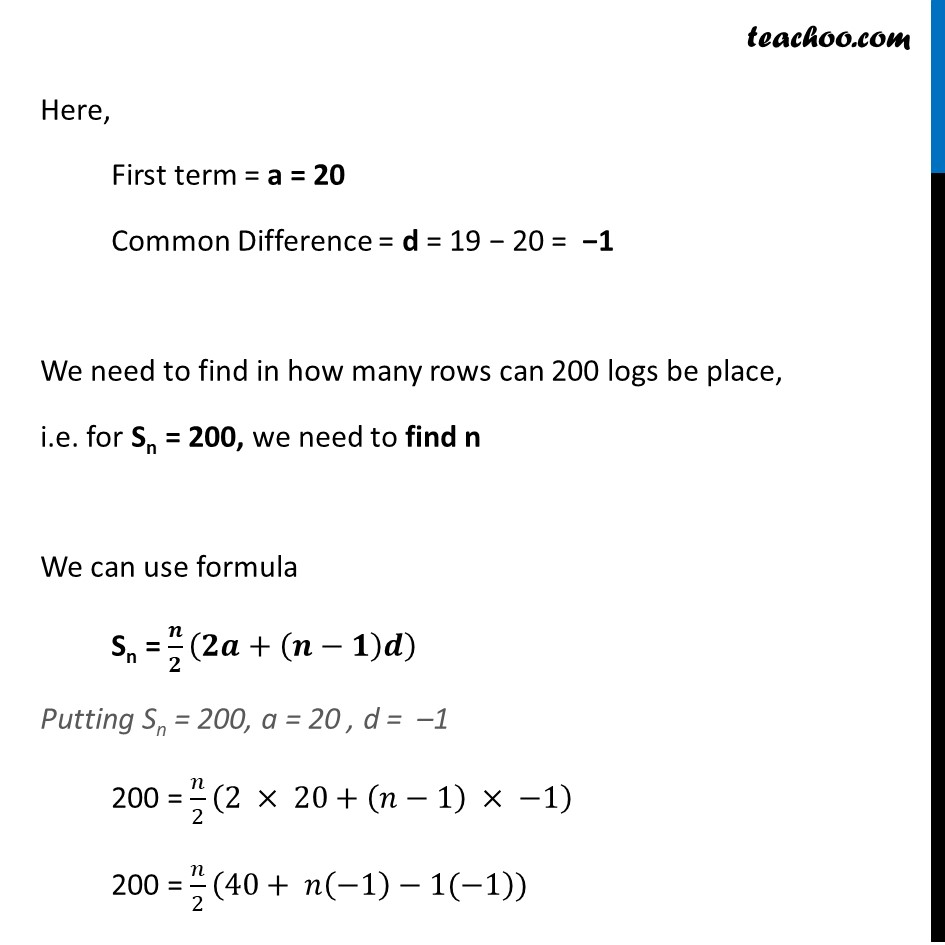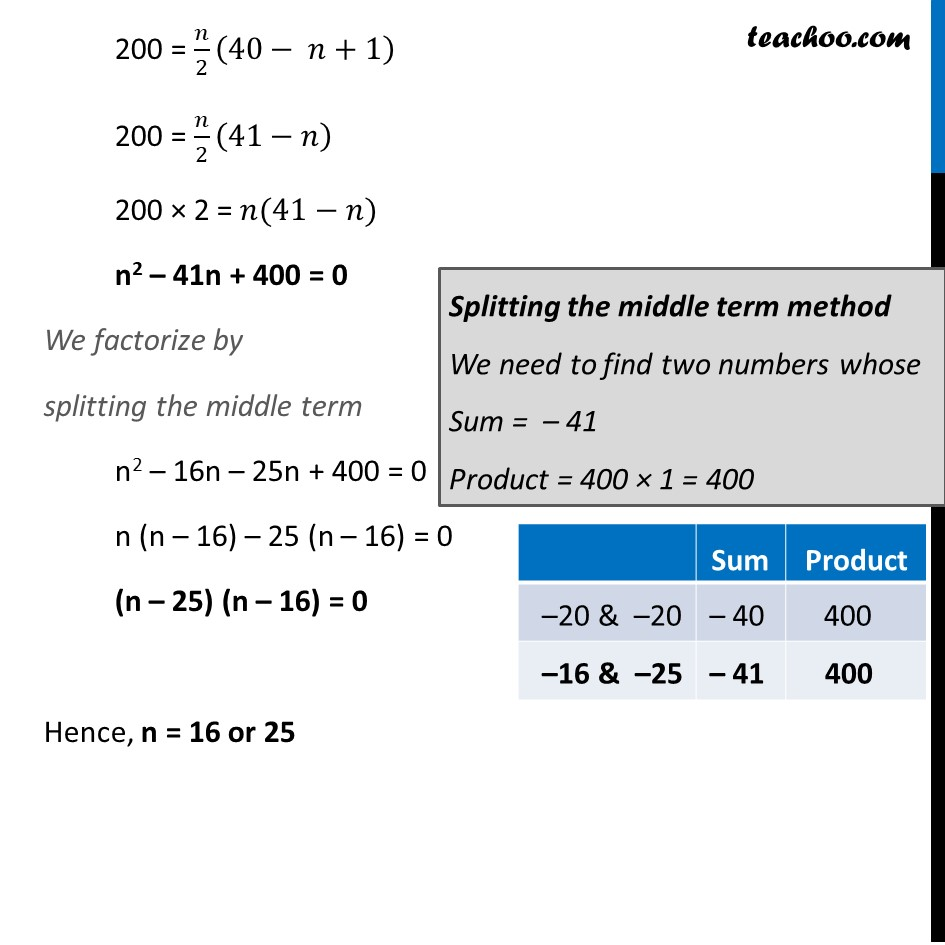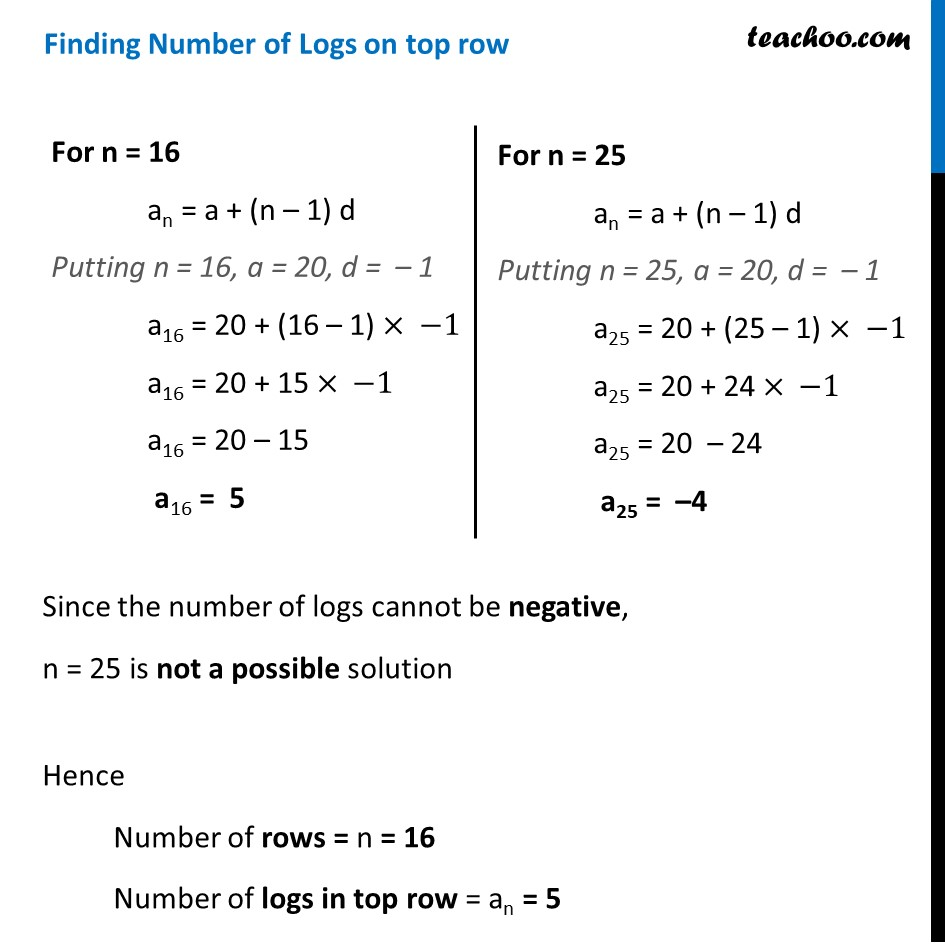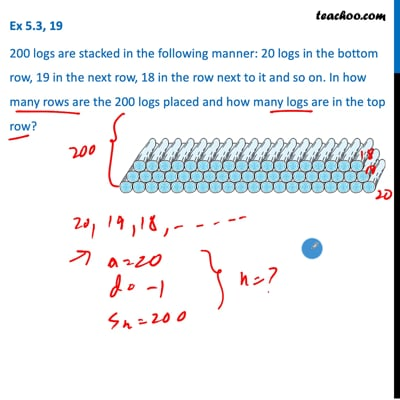This video is only available for Teachoo black users

Solve all your doubts with Teachoo Black (new monthly pack available now!)

### Transcript

Ex 5.3, 19 200 logs are stacked in the following manner: 20 logs in the bottom row, 19 in the next row, 18 in the row next to it and so on. In how many rows are the 200 logs placed and how many logs are in the top row? Number of logs in 1st row = 20 Number of logs in 2nd row = 19 Number of logs in 3rd row = 18 Hence the series is 20, 19, 18, …….. Since difference is same, it is an AP Here, First term = a = 20 Common Difference = d = 19 − 20 = −1 We need to find in how many rows can 200 logs be place, i.e. for Sn = 200, we need to find n We can use formula Sn = 𝒏/𝟐 (𝟐𝒂+(𝒏−𝟏)𝒅) Putting Sn = 200, a = 20 , d = –1 200 = 𝑛/2 (2 × 20+(𝑛−1) × −1) 200 = 𝑛/2 (40+ 𝑛(−1)−1(−1)) 200 = 𝑛/2 (40− 𝑛+1) 200 = 𝑛/2 (41−𝑛) 200 × 2 = 𝑛(41−𝑛) n2 – 41n + 400 = 0 We factorize by splitting the middle term n2 – 16n – 25n + 400 = 0 n (n – 16) – 25 (n – 16) = 0 (n – 25) (n – 16) = 0 Hence, n = 16 or 25 Splitting the middle term method We need to find two numbers whose Sum = – 41 Product = 400 × 1 = 400 Finding Number of Logs on top row For n = 16 an = a + (n – 1) d Putting n = 16, a = 20, d = – 1 a16 = 20 + (16 – 1) × −1 a16 = 20 + 15 × −1 a16 = 20 – 15 a16 = 5 For n = 25 an = a + (n – 1) d Putting n = 25, a = 20, d = – 1 a25 = 20 + (25 – 1) × −1 a25 = 20 + 24 × −1 a25 = 20 – 24 a25 = –4 Since the number of logs cannot be negative, n = 25 is not a possible solution Hence Number of rows = n = 16 Number of logs in top row = an = 5